Скачать презентацию MBA 201 A Section Intro 4 Introduction

cd30dbebcd446e4979f2118dd5db4ede.ppt

• Количество слайдов: 16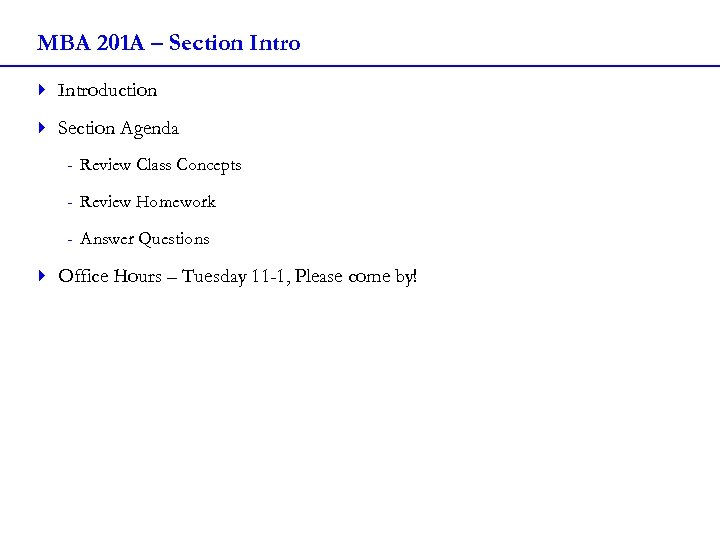MBA 201 A – Section Intro 4 Introduction 4 Section Agenda - Review Class Concepts - Review Homework - Answer Questions 4 Office Hours – Tuesday 11 -1, Please come by!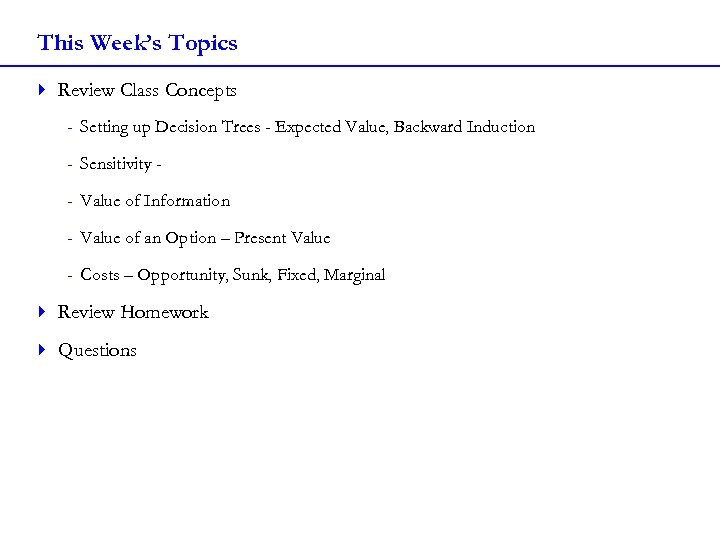This Week’s Topics 4 Review Class Concepts - Setting up Decision Trees - Expected Value, Backward Induction - Sensitivity - Value of Information - Value of an Option – Present Value - Costs – Opportunity, Sunk, Fixed, Marginal 4 Review Homework 4 Questions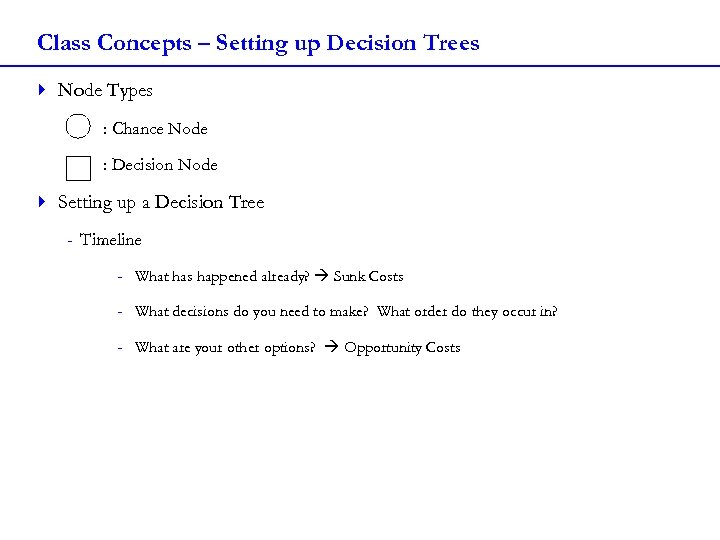Class Concepts – Setting up Decision Trees 4 Node Types : Chance Node : Decision Node 4 Setting up a Decision Tree - Timeline - What has happened already? Sunk Costs - What decisions do you need to make? What order do they occur in? - What are your other options? Opportunity Costs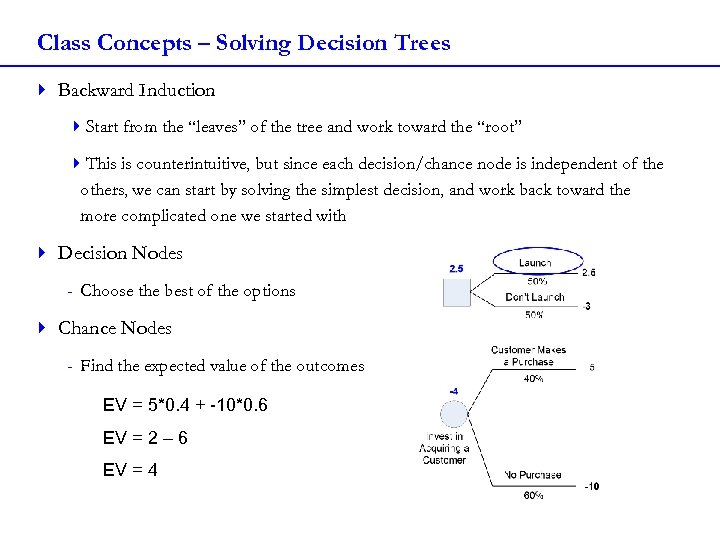Class Concepts – Solving Decision Trees 4 Backward Induction 4 Start from the “leaves” of the tree and work toward the “root” 4 This is counterintuitive, but since each decision/chance node is independent of the others, we can start by solving the simplest decision, and work back toward the more complicated one we started with 4 Decision Nodes - Choose the best of the options 4 Chance Nodes - Find the expected value of the outcomes EV = 5*0. 4 + -10*0. 6 EV = 2 – 6 EV = 4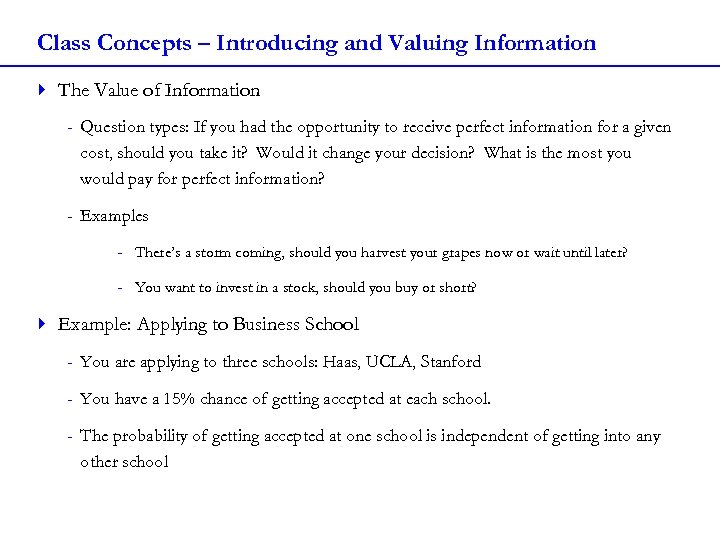Class Concepts – Introducing and Valuing Information 4 The Value of Information - Question types: If you had the opportunity to receive perfect information for a given cost, should you take it? Would it change your decision? What is the most you would pay for perfect information? - Examples - There’s a storm coming, should you harvest your grapes now or wait until later? - You want to invest in a stock, should you buy or short? 4 Example: Applying to Business School - You are applying to three schools: Haas, UCLA, Stanford - You have a 15% chance of getting accepted at each school. - The probability of getting accepted at one school is independent of getting into any other school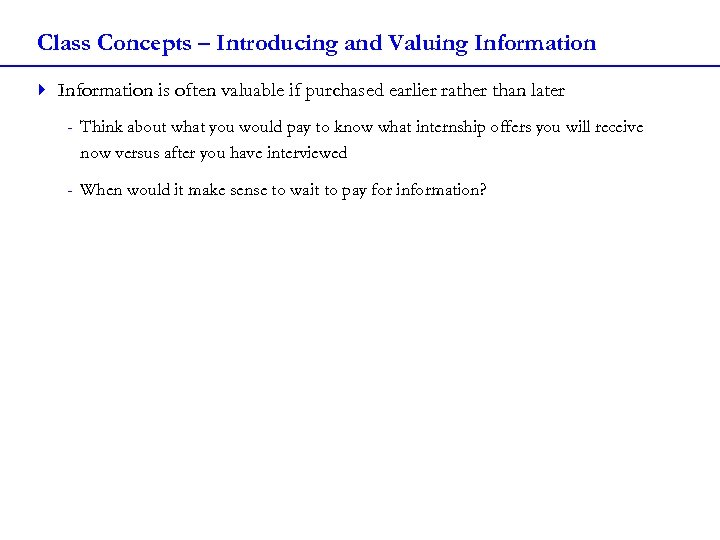Class Concepts – Introducing and Valuing Information 4 Information is often valuable if purchased earlier rather than later - Think about what you would pay to know what internship offers you will receive now versus after you have interviewed - When would it make sense to wait to pay for information?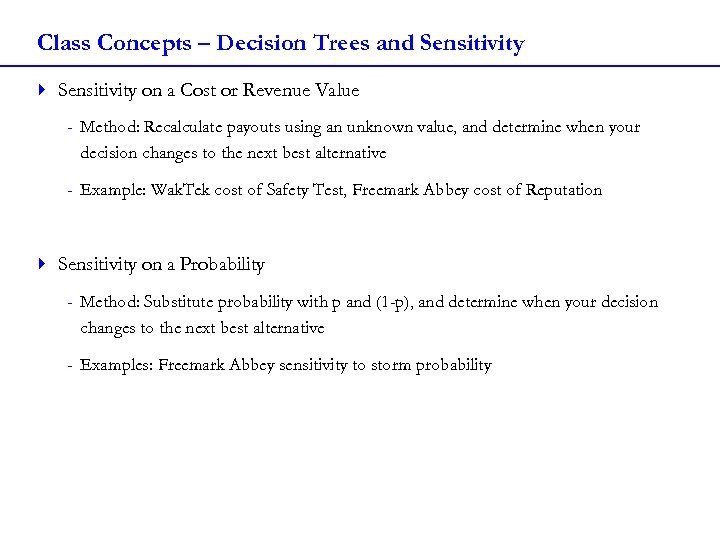Class Concepts – Decision Trees and Sensitivity 4 Sensitivity on a Cost or Revenue Value - Method: Recalculate payouts using an unknown value, and determine when your decision changes to the next best alternative - Example: Wak. Tek cost of Safety Test, Freemark Abbey cost of Reputation 4 Sensitivity on a Probability - Method: Substitute probability with p and (1 -p), and determine when your decision changes to the next best alternative - Examples: Freemark Abbey sensitivity to storm probability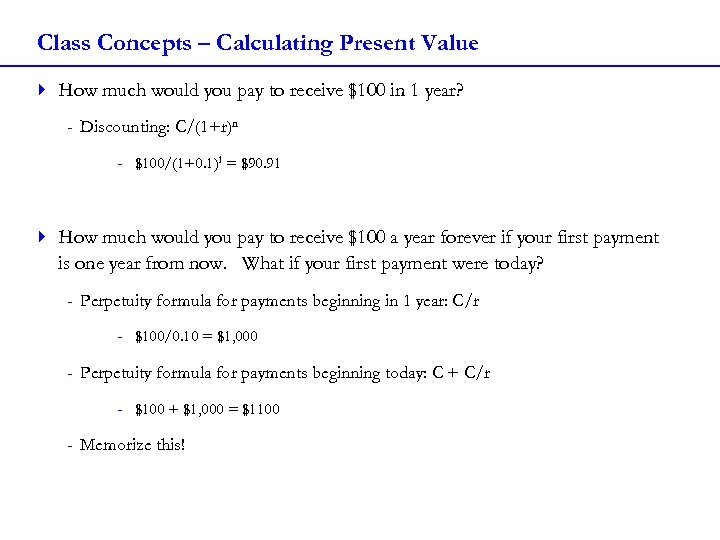Class Concepts – Calculating Present Value 4 How much would you pay to receive \$100 in 1 year? - Discounting: C/(1+r)n - \$100/(1+0. 1)1 = \$90. 91 4 How much would you pay to receive \$100 a year forever if your first payment is one year from now. What if your first payment were today? - Perpetuity formula for payments beginning in 1 year: C/r - \$100/0. 10 = \$1, 000 - Perpetuity formula for payments beginning today: C + C/r - \$100 + \$1, 000 = \$1100 - Memorize this!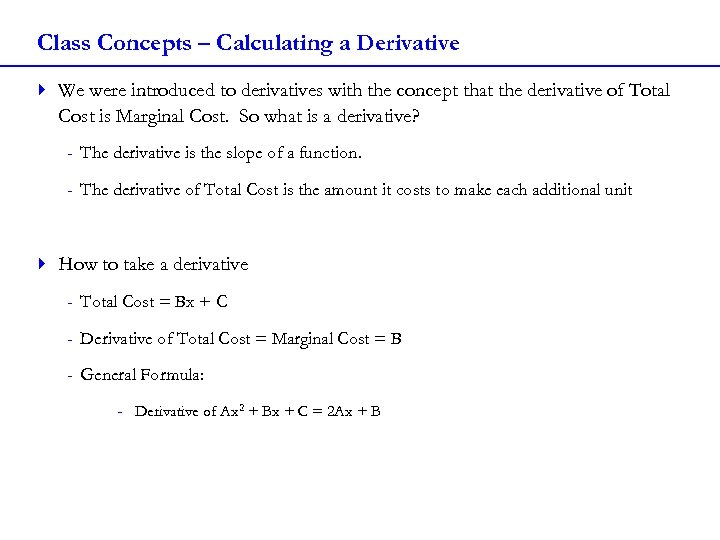Class Concepts – Calculating a Derivative 4 We were introduced to derivatives with the concept that the derivative of Total Cost is Marginal Cost. So what is a derivative? - The derivative is the slope of a function. - The derivative of Total Cost is the amount it costs to make each additional unit 4 How to take a derivative - Total Cost = Bx + C - Derivative of Total Cost = Marginal Cost = B - General Formula: - Derivative of Ax 2 + Bx + C = 2 Ax + B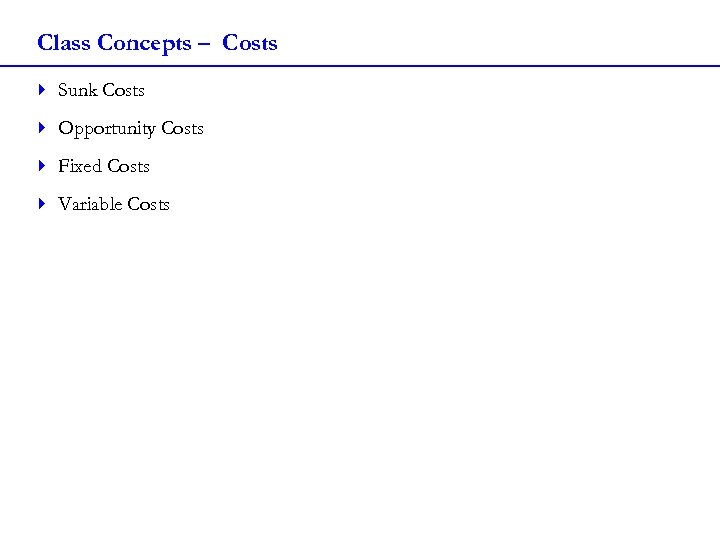Class Concepts – Costs 4 Sunk Costs 4 Opportunity Costs 4 Fixed Costs 4 Variable CostsHomework – Wak. Tek – Question 2 a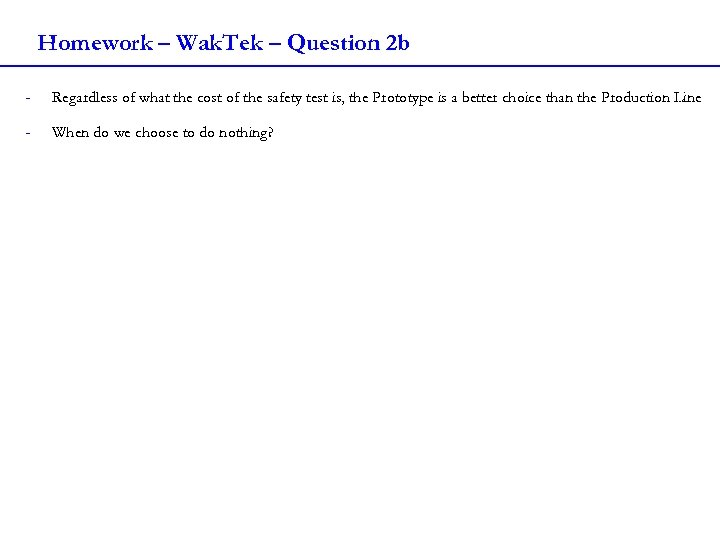Homework – Wak. Tek – Question 2 b - Regardless of what the cost of the safety test is, the Prototype is a better choice than the Production Line - When do we choose to do nothing?Homework – Wak. Tek – Question 2 c - Simultaneous Information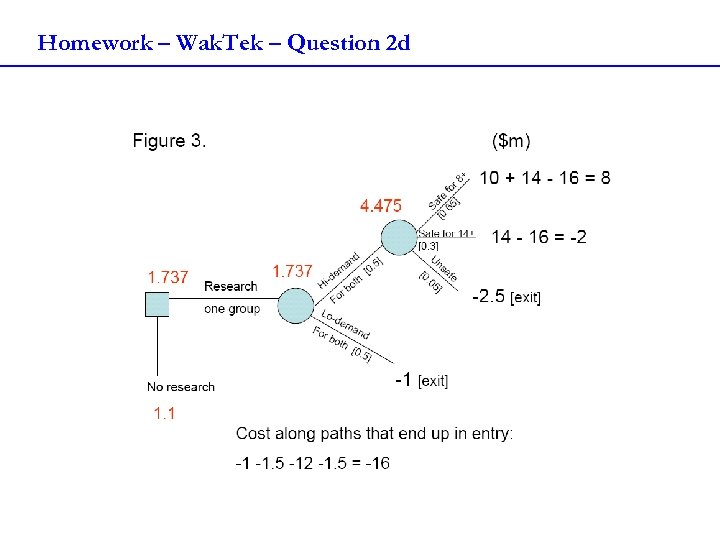Homework – Wak. Tek – Question 2 d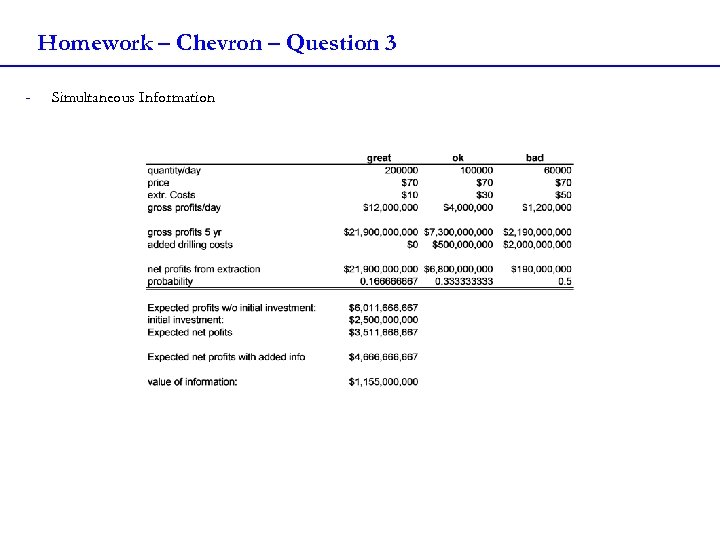Homework – Chevron – Question 3 - Simultaneous Information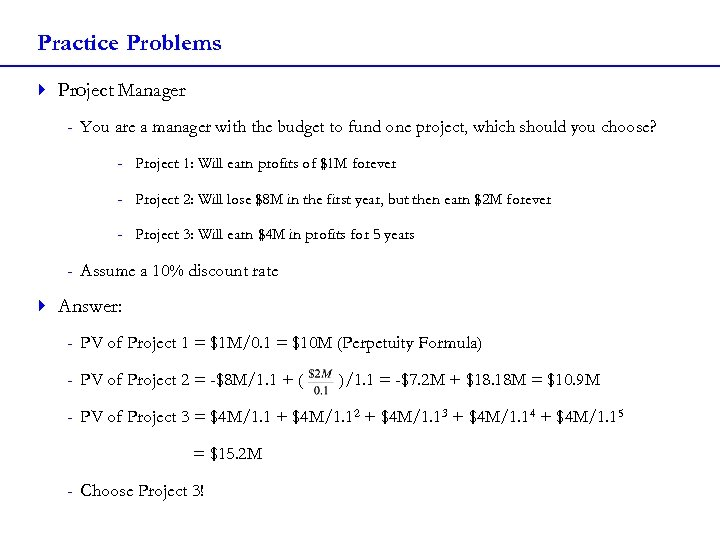Practice Problems 4 Project Manager - You are a manager with the budget to fund one project, which should you choose? - Project 1: Will earn profits of \$1 M forever - Project 2: Will lose \$8 M in the first year, but then earn \$2 M forever - Project 3: Will earn \$4 M in profits for 5 years - Assume a 10% discount rate 4 Answer: - PV of Project 1 = \$1 M/0. 1 = \$10 M (Perpetuity Formula) - PV of Project 2 = -\$8 M/1. 1 + ( )/1. 1 = -\$7. 2 M + \$18. 18 M = \$10. 9 M - PV of Project 3 = \$4 M/1. 1 + \$4 M/1. 12 + \$4 M/1. 13 + \$4 M/1. 14 + \$4 M/1. 15 = \$15. 2 M - Choose Project 3!